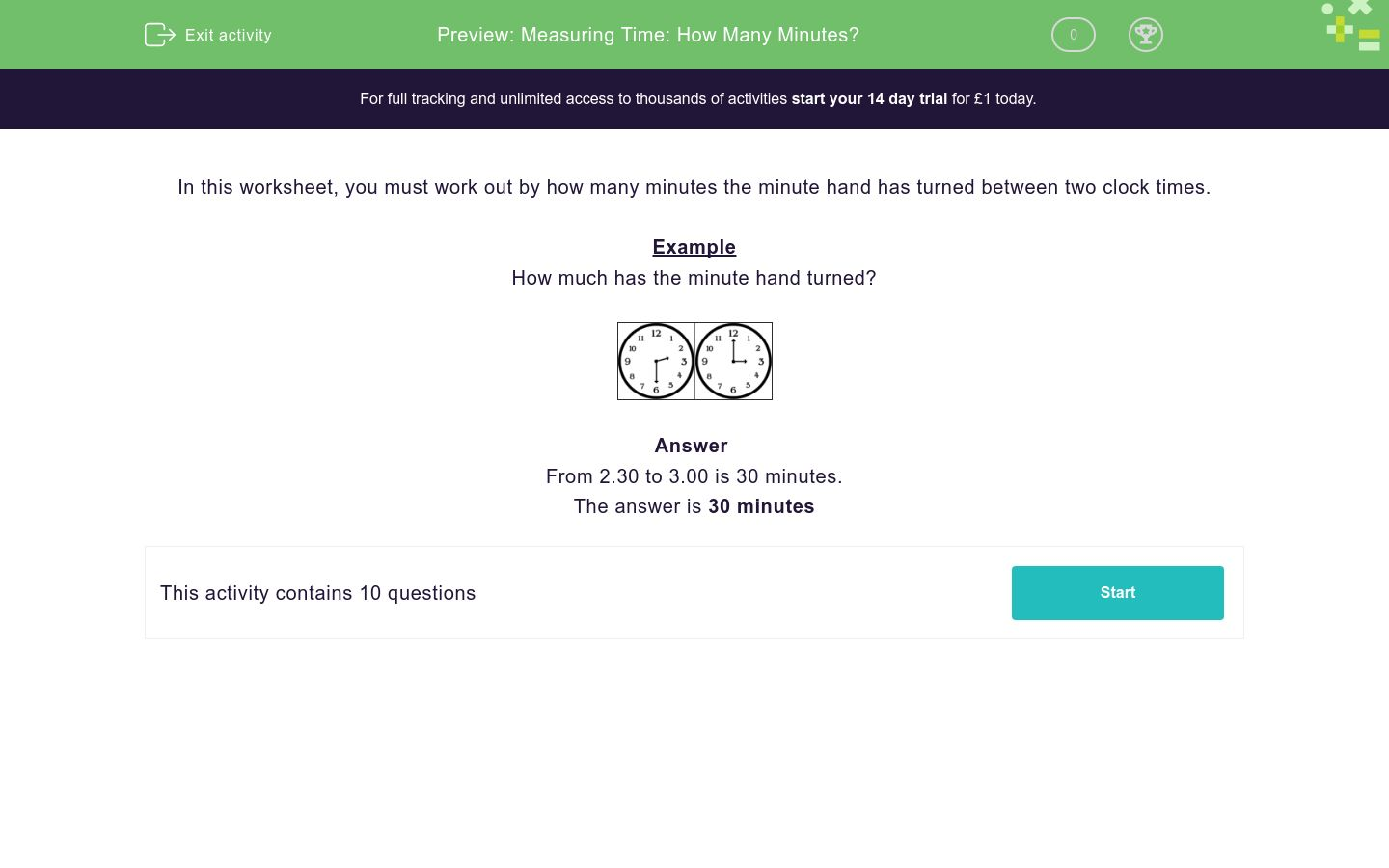Measuring Time: How Many Minutes?

In this worksheet, students work out by how many minutes the minute hand has turned between two clock times.Key stage:  KS 1

Curriculum topic:  Measurement

Curriculum subtopic:  Compare/Sequence Time Intervals

Difficulty level:QUESTION 1 of 10

In this worksheet, you must work out by how many minutes the minute hand has turned between two clock times.

Example

How much has the minute hand turned?From 2.30 to 3.00 is 30 minutes.

How much has the minute hand turned?30 minutes

15 minutes

45 minutes

How much has the minute hand turned?30 minutes

15 minutes

45 minutes

How much has the minute hand turned?30 minutes

15 minutes

45 minutes

How much has the minute hand turned?30 minutes

15 minutes

45 minutes

How much has the minute hand turned?30 minutes

15 minutes

45 minutes

How much has the minute hand turned?30 minutes

15 minutes

45 minutes

How much has the minute hand turned?30 minutes

15 minutes

45 minutes

How much has the minute hand turned?30 minutes

15 minutes

45 minutes

How much has the minute hand turned?30 minutes

15 minutes

45 minutes

How much has the minute hand turned?30 minutes

15 minutes

45 minutes

• Question 1

How much has the minute hand turned?15 minutes
• Question 2

How much has the minute hand turned?30 minutes
• Question 3

How much has the minute hand turned?45 minutes
• Question 4

How much has the minute hand turned?30 minutes
• Question 5

How much has the minute hand turned?15 minutes
• Question 6

How much has the minute hand turned?30 minutes
• Question 7

How much has the minute hand turned?30 minutes
• Question 8

How much has the minute hand turned?45 minutes
• Question 9

How much has the minute hand turned?30 minutes
• Question 10

How much has the minute hand turned?